# 5 Real-world Examples of Logistic Regression Application## Overview: what is logistic regression

Logistic regression is a machine learning method used in the classification problem when you need to distinguish one class from another. The simplest case is a binary classification. This is like a question that we can answer with either “yes” or “no.” We only have two classes: a positive class and negative class. Usually, a positive class points to the presence of some entity while negative class points to the absence of it.

In this case, we need to predict a single value - the probability that entity is present. To do so, it will be good for us to have a function that maps any real value to value in the interval between 0 and 1.

Let’s look at this function plot.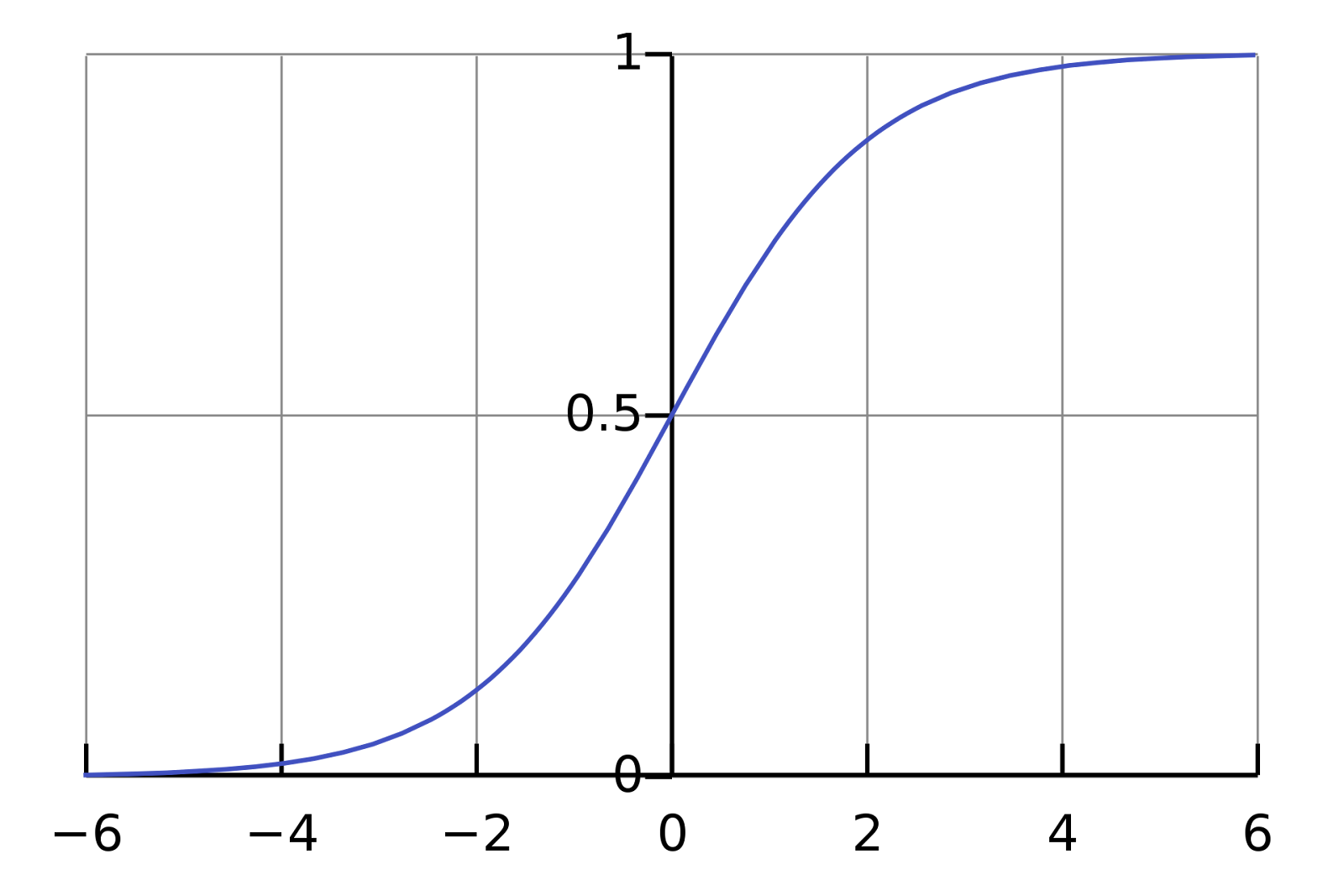It shows a pretty decent mapping between R and the (0, 1) interval. It suits our requirements.

This is the so-called sigmoid function and it is defined this way: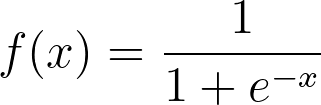Most far from 0 values of x are mapped close to 0 or close to 1 values of y. Values close to 0 of x will be a good approximation of probability in our algorithm. Then we can choose a threshold value and transform probability to 0 or 1 prediction.

Sigmoid is an activation function for logistic regression. Now let’s define the cost function for our optimization algorithm.

The first thing that comes into mind when we think about cost function is a classic square error function.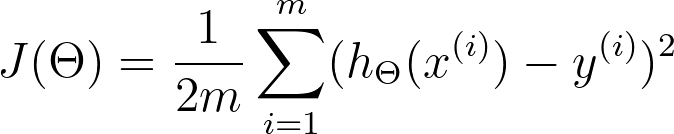Where

m - number of examples,

x(i) - feature vector for i-th example,

y(i) - actual value for i-th example,

θ  - parameters vector.

If we have a linear activation function hθ(x) then it’s okay. But with our new sigmoid function, we have no positive second derivative for square error. It means that it is not convex. We don’t want to stuck in local optima, thus we define a new cost function: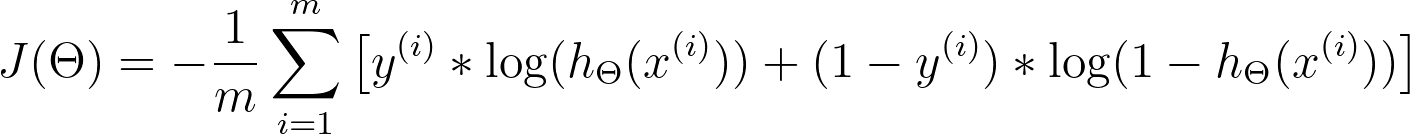This is called a cross-entropy cost. If you look carefully, you may notice that when a prediction is close to actual value then cost will be close to zero for both 0 and 1 actual values.

Let's suppose we have features x1,x2 ,....,xn, and y value for every entity.

Then we have n+1-dimensioned θ parameters vector, such that: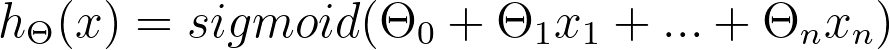And we optimize θ with gradient descent and cross-entropy cost. That’s it!

## Why logistic regression is cool

Logistic regression is simpler than modern deep learning algorithms, but simpler algorithms don't mean worse. There are many cases where logistic regression is more than enough. It also has advantages that are very significant in real cases.

First of all, it’s very simple to use. Logistic regression is realized in many statistical packages such as SAS, STATISTICA, R packages, and other tools. This makes it easy to use even if you do not have an advanced machine learning team for your task.

The second advantage is speed, and sometimes this is crucial.

Lastly, the most significant advantage of logistic regression over neural networks is transparency. Neural networks work as a black box - you never know why it makes one or another decision. There are a lot of highly regulated industries where this approach is not acceptable. Logistic regression, in contrast, may be called the “white box”. You always know why you rejected a loan application or why your patient’s diagnosis looks good or bad. That is what we’ll talk about in detail.

## 5 real-world cases where logistic regression was effectively used

### Credit scoring

ID Finance is a financial company that makes predictive models for credit scoring. They need their models to be easily interpretable. They can be asked by a regulator about a certain decision at any moment.

Data preprocessing for credit scoring modeling includes such a step like reducing correlated variables. It’s difficult if you have more than 15 variables in your model. For logistic regression, it is easy to find out which variables affect the final result of the predictions more and which ones less. It is also possible to find the optimal number of features and eliminate redundant variables with methods like recursive feature elimination.

At the final step, they can export prediction results to an Excel file, and analytic even without technical skills can get insights from this data.

At some point, ID finance refused the use of third-party statistical applications and rewrote their algorithms for building models in Python. This has led to a significant increase in the speed of model development. But they did not abandon logistic regression in favor of more complex algorithms. Logistic regression is widely used in credit scoring and it shows remarkable results.

### Medicine

Medical information is gathered in such a way that when a research group studies a biological molecule and its properties, they publish a paper about it. Thus, there is a huge amount of medical data about various compounds, but they are not combined into a single database.

Miroculus is a company that develops express blood test kits. Its goal is to identify diseases that are affected by genes, such as oncology diseases. The company entered into an agreement with Microsoft to develop an algorithm to identify the relationship between certain micro-RNA and genes.

The developers used a database of scientific articles and applied text analysis methods to obtain feature vectors. The text was split into the sentences, the entities were extracted, labeled data generated from known relations, and after several other text transformation methods, each sentence was converted into a 200-dimensional vector.

After converting the text and extracting the distinguishing features, a classification was made for the presence of a link between microRNA and a certain gene. Algorithms such as logistic regression, support vector machine, and random forest were considered as models. Logistic regression was selected because it demonstrated the best results in speed and accuracy.

Logistic regression is well suited for this data type when we need to predict a binary answer. Is there a connection between the elements or not? Thanks to this algorithm, the accuracy of a quick blood test have been increased.

### Text editing

As we talked about texts, it is worth mentioning that logistic regression is a popular choice in many natural language processing tasks. First, the text preprocessing is performed, then features are extracted, and finally, logistic regression is used to make some claim about a text fragment. Toxic speech detection, topic classification for questions to support, and email sorting are examples where logistic regression shows good results. Other popular algorithms for making a decision in these fields are support vector machines and random forest.

Let's look at the less popular NLP task - text transformation or digitalization. One company has faced this problem: they had a lot of PDF text files and texts extracted from scans with the OCR system. Such files had a fixed structure with line break by the characters of the end of the paragraph, and with hyphens. They needed to transform this data into usable text with grammatical and semantic correct formatting.

The developer manually marked out three large documents, adding special characters to the beginning of the line indicating whether it should be glued to the previous line. As features were chosen: the length of the current and previous lines in characters, the average length of several lines around, whether the last character of the previous line is a letter or a digit, punctuation mark on which the previous line ends, and some other properties. All string and boolean features were transformed into numerical. Then logistic regression was trained.

It showed a few errors and these were mainly the same errors that humans can make in such a situation. There were very few easy human-readable errors. Logistic regression showed excellent results in this task, and a lot of texts were automatically transformed using this method.

### Hotel Booking

Booking.com has a lot of machine learning methods literally everywhere on the site. They try to predict users' intentions and recognize entities. Where will you go, where do you prefer to stop, what are you planning to do? Some predictions are made even if the user didn't type anything in the search line yet. But how did they start to do this? No one can build a huge and complex system with various machine learning algorithms from scratch. They have accumulated some statistics and created some simple models as the first steps.

Most of the features at such services like booking.com are rather categorical than numerical. Sometimes it becomes necessary to predict an event without specific data about the user. For example, all the data they have is where the user is from and where she wants to go. Logistic regression is ideal for such needs.

Here is a histogram of logistic regression trying to predict either user will change a journey date or not. It was presented at HighLoad++ Siberia conference in 2018.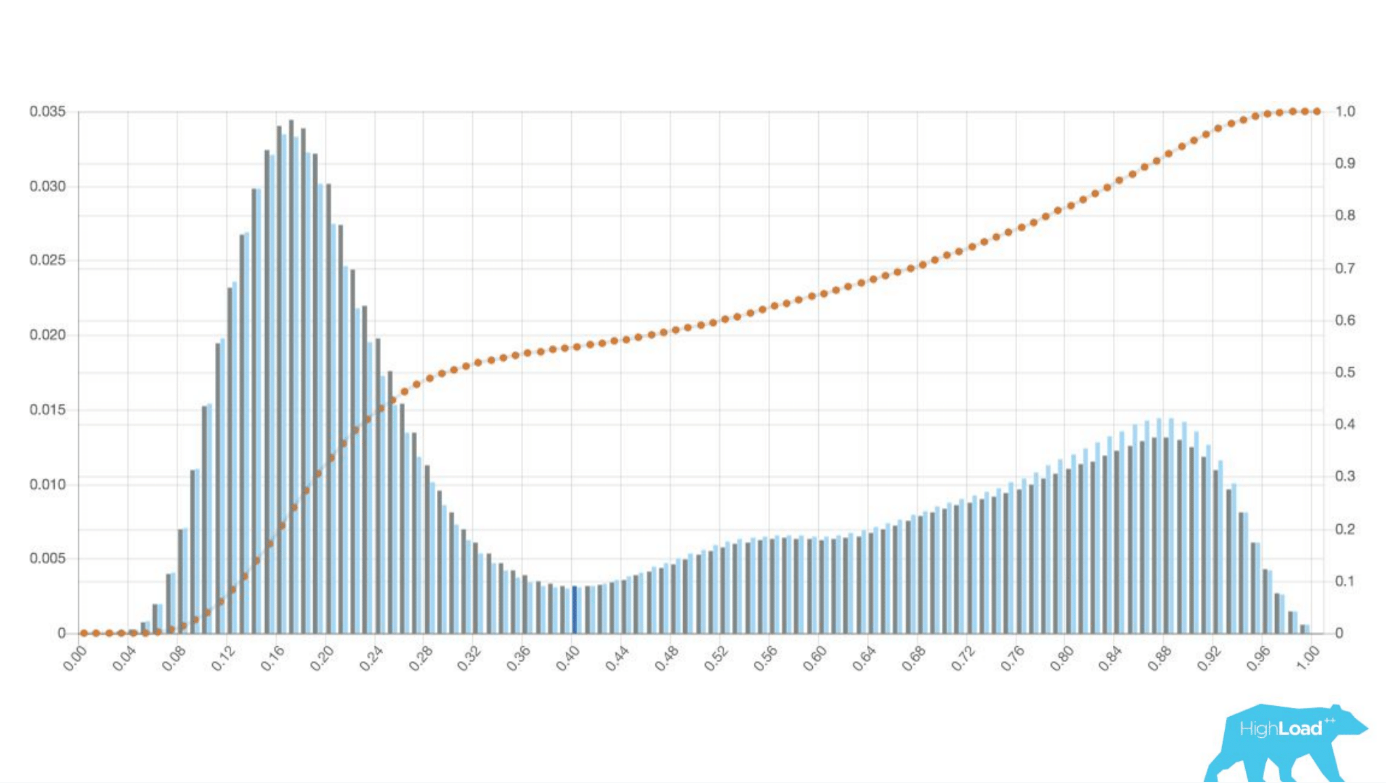Logistic regression could well separate two classes of users. Based on this data, the company then can decide if it will change an interface for one class of users.

You probably saw this functionality if you have used Booking. Now you know there is logistic regression somewhere behind this application.

### Gaming

Speed is one of the advantages of logistic regression, and it is extremely useful in the gaming industry. Speed is very important in a game. Very popular today are the games where you can use in-game purchases to improve the gaming qualities of your character, or for fancy appearance and communication with other players. In-game purchases are a good place to introduce a recommendation system.
Tencent is the world's largest gaming company. It uses such systems to suggest gamers' equipment which they would like to buy. Their algorithm analyzes a very large amount of data about user behavior and gives suggestions about equipment a particular user may want to acquire on the run. This algorithm is logistic regression.
There are three types of recommendation systems. The collaborative system predicts what the user would like to buy based on ratings from users with similar preferences in previous purchases, and other activity. A content-based algorithm makes its decision based on properties specified in the item description and what the user indicated as interests in her profile. The third type is the hybrid and it is a combination of two previous types.

Both the description and the preferences of other users can be used as features in logistic regression. You only need to transform them into a similar format and normalize. Logistic regression will work fast and show good results.

## Conclusion

Logistic regression is one of the classic machine learning methods. It forms a basis of machine learning along with linear regression, k-mean clustering, principal component analysis, and some others. Neural networks were developed on top of logistic regression. You can successfully use logistic regression in your tasks even if you are not a machine learning specialist. But it is very unlikely that someone can become a good machine learning specialist without knowledge of logistic regression.